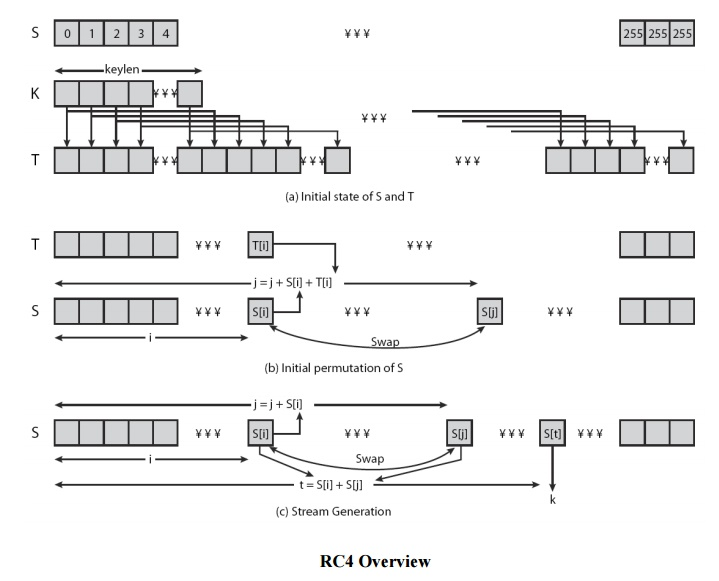# RC4

1. RC4 Key Schedule 2. RC4 Encryption 3 RC4 Security

RC4

o   a proprietary cipher owned by RSA DSI

o   another Ron Rivest design, simple but effective

o   variable key size, byte-oriented stream cipher

o   widely used (web SSL/TLS, wireless WEP)

o   key forms random permutation of all 8-bit values

o   uses that permutation to scramble input info processed a byte at a time

1. RC4 Key Schedule

·        starts with an array S of numbers: 0..255

·        use key to well and truly shuffle

·        S forms internal state of the cipher

for i = 0 to 255 do S[i] = i

T[i] = K[i mod keylen])

j = 0

for i = 0 to 255 do

j = (j + S[i] + T[i]) (mod 256)

swap (S[i], S[j])

2. RC4 Encryption

·        encryption continues shuffling array values

·        sum of shuffled pair selects "stream key" value from permutation

·        XOR S[t] with next byte of message to en/decrypt

i = j = 0

for each message byte Mi

i = (i + 1) (mod 256)

j = (j + S[i]) (mod 256) swap(S[i], S[j])

t = (S[i] + S[j]) (mod 256)

Ci = Mi XOR S[t]3 RC4 Security

·        claimed secure against known attacks ü have some analyses, none practical

·        result is very non-linear

·        since RC4 is a stream cipher, must never reuse a key

·        have a concern with WEP, but due to key handling rather than RC4 itself.

Study Material, Lecturing Notes, Assignment, Reference, Wiki description explanation, brief detail
Cryptography and Network Security : RC4 |